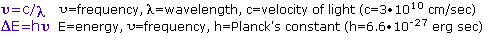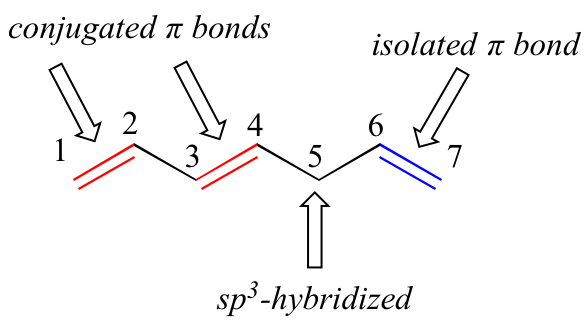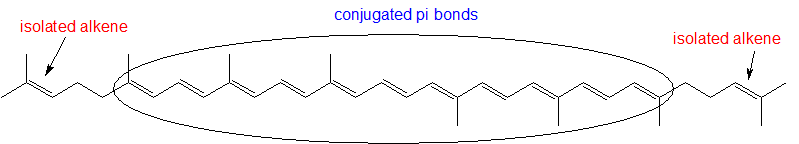# 15.9: Structure Determination in Conjugated Systems - Ultraviolet Spectroscopy

$$\newcommand{\vecs}{\overset { \rightharpoonup} {\mathbf{#1}} }$$ $$\newcommand{\vecd}{\overset{-\!-\!\rightharpoonup}{\vphantom{a}\smash {#1}}}$$$$\newcommand{\id}{\mathrm{id}}$$ $$\newcommand{\Span}{\mathrm{span}}$$ $$\newcommand{\kernel}{\mathrm{null}\,}$$ $$\newcommand{\range}{\mathrm{range}\,}$$ $$\newcommand{\RealPart}{\mathrm{Re}}$$ $$\newcommand{\ImaginaryPart}{\mathrm{Im}}$$ $$\newcommand{\Argument}{\mathrm{Arg}}$$ $$\newcommand{\norm}{\| #1 \|}$$ $$\newcommand{\inner}{\langle #1, #2 \rangle}$$ $$\newcommand{\Span}{\mathrm{span}}$$ $$\newcommand{\id}{\mathrm{id}}$$ $$\newcommand{\Span}{\mathrm{span}}$$ $$\newcommand{\kernel}{\mathrm{null}\,}$$ $$\newcommand{\range}{\mathrm{range}\,}$$ $$\newcommand{\RealPart}{\mathrm{Re}}$$ $$\newcommand{\ImaginaryPart}{\mathrm{Im}}$$ $$\newcommand{\Argument}{\mathrm{Arg}}$$ $$\newcommand{\norm}{\| #1 \|}$$ $$\newcommand{\inner}{\langle #1, #2 \rangle}$$ $$\newcommand{\Span}{\mathrm{span}}$$$$\newcommand{\AA}{\unicode[.8,0]{x212B}}$$

## The Electromagnetic Spectrum

The visible spectrum constitutes but a small part of the total radiation spectrum. Most of the radiation that surrounds us cannot be seen, but can be detected by dedicated sensing instruments. This electromagnetic spectrum ranges from very short wavelengths (including gamma and x-rays) to very long wavelengths (including microwaves and broadcast radio waves). The following chart displays many of the important regions of this spectrum, and demonstrates the inverse relationship between wavelength and frequency (shown in the top equation below the chart).The energy associated with a given segment of the spectrum is proportional to its frequency. The bottom equation describes this relationship, which provides the energy carried by a photon of a given wavelength of radiation.To obtain specific frequency, wavelength and energy values use this calculator.• Indigo: 420 - 440 nm
• Green: 490 - 570 nm
• Orange: 585 - 620 nm
• Red: 620 - 780 nm

When white light passes through or is reflected by a colored substance, a characteristic portion of the mixed wavelengths is absorbed. The remaining light will then assume the complementary color to the wavelength(s) absorbed. This relationship is demonstrated by the color wheel shown below. Here, complementary colors are diametrically opposite each other. Thus, absorption of 420-430 nm light renders a substance yellow, and absorption of 500-520 nm light makes it red. Green is unique in that it can be created by absoption close to 400 nm as well as absorption near 800 nm.Early humans valued colored pigments, and used them for decorative purposes. Many of these were inorganic minerals, but several important organic dyes were also known. These included the crimson pigment, kermesic acid, the blue dye, indigo, and the yellow saffron pigment, crocetin. A rare dibromo-indigo derivative, punicin, was used to color the robes of the royal and wealthy. The deep orange hydrocarbon carotene is widely distributed in plants, but is not sufficiently stable to be used as permanent pigment, other than for food coloring. A common feature of all these colored compounds, displayed below, is a system of extensively conjugated $$\pi$$-electrons.

## UV-Visible Absorption Spectra

To understand why some compounds are colored and others are not, and to determine the relationship of conjugation to color, we must make accurate measurements of light absorption at different wavelengths in and near the visible part of the spectrum. Commercial optical spectrometers enable such experiments to be conducted with ease, and usually survey both the near ultraviolet and visible portions of the spectrum.

The visible region of the spectrum comprises photon energies of 36 to 72 kcal/mole, and the near ultraviolet region, out to 200 nm, extends this energy range to 143 kcal/mole. Ultraviolet radiation having wavelengths less than 200 nm is difficult to handle, and is seldom used as a routine tool for structural analysis.The energies noted above are sufficient to promote or excite a molecular electron to a higher energy orbital. Consequently, absorption spectroscopy carried out in this region is sometimes called "electronic spectroscopy". A diagram showing the various kinds of electronic excitation that may occur in organic molecules is shown on the left. Of the six transitions outlined, only the two lowest energy ones (left-most, colored blue) are achieved by the energies available in the 200 to 800 nm spectrum. As a rule, energetically favored electron promotion will be from the highest occupied molecular orbital (HOMO) to the lowest unoccupied molecular orbital (LUMO), and the resulting species is called an excited state.

When sample molecules are exposed to light having an energy that matches a possible electronic transition within the molecule, some of the light energy will be absorbed as the electron is promoted to a higher energy orbital. An optical spectrometer records the wavelengths at which absorption occurs, together with the degree of absorption at each wavelength. The resulting spectrum is presented as a graph of absorbance (A) versus wavelength, as in the isoprene spectrum shown below. Since isoprene is colorless, it does not absorb in the visible part of the spectrum and this region is not displayed on the graph. Absorbance usually ranges from 0 (no absorption) to 2 (99% absorption), and is precisely defined in context with spectrometer operation.

## Electronic transitions

Let’s take as our first example the simple case of molecular hydrogen, H2. As you may recall from section 2.1A, the molecular orbital picture for the hydrogen molecule consists of one bonding σ MO, and a higher energy antibonding σ* MO. When the molecule is in the ground state, both electrons are paired in the lower-energy bonding orbital – this is the Highest Occupied Molecular Orbital (HOMO). The antibonding σ* orbital, in turn, is the Lowest Unoccupied Molecular Orbital (LUMO).If the molecule is exposed to light of a wavelength with energy equal to ΔE, the HOMO-LUMO energy gap, this wavelength will be absorbed and the energy used to bump one of the electrons from the HOMO to the LUMO – in other words, from the σ to the σ* orbital. This is referred to as a σ - σ* transition. ΔE for this electronic transition is 258 kcal/mol, corresponding to light with a wavelength of 111 nm.

When a double-bonded molecule such as ethene (common name ethylene) absorbs light, it undergoes a π - π* transition. Because π- π* energy gaps are narrower than σ - σ* gaps, ethene absorbs light at 165 nm - a longer wavelength than molecular hydrogen.The electronic transitions of both molecular hydrogen and ethene are too energetic to be accurately recorded by standard UV spectrophotometers, which generally have a range of 220 – 700 nm. Where UV-vis spectroscopy becomes useful to most organic and biological chemists is in the study of molecules with conjugated pi systems. In these groups, the energy gap for π -π* transitions is smaller than for isolated double bonds, and thus the wavelength absorbed is longer. Molecules or parts of molecules that absorb light strongly in the UV-vis region are called chromophores.

Next, we'll consider the 1,3-butadiene molecule. From valence orbital theory alone we might expect that the C2-C3 bond in this molecule, because it is a sigma bond, would be able to rotate freely.Experimentally, however, it is observed that there is a significant barrier to rotation about the C2-C3 bond, and that the entire molecule is planar. In addition, the C2-C3 bond is 148 pm long, shorter than a typical carbon-carbon single bond (about 154 pm), though longer than a typical double bond (about 134 pm).

Molecular orbital theory accounts for these observations with the concept of delocalized π bonds. In this picture, the four p atomic orbitals combine mathematically to form four pi molecular orbitals of increasing energy. Two of these - the bonding pi orbitals - are lower in energy than the p atomic orbitals from which they are formed, while two - the antibonding pi orbitals - are higher in energy.The lowest energy molecular orbital, pi1, has only constructive interaction and zero nodes. Higher in energy, but still lower than the isolated p orbitals, the pi2 orbital has one node but two constructive interactions - thus it is still a bonding orbital overall. Looking at the two antibonding orbitals, pi3* has two nodes and one constructive interaction, while pi4* has three nodes and zero constructive interactions.

By the aufbau principle, the four electrons from the isolated 2pz atomic orbitals are placed in the bonding pi1 and pi2 MO’s. Because pi1 includes constructive interaction between C2 and C3, there is a degree, in the 1,3-butadiene molecule, of pi-bonding interaction between these two carbons, which accounts for its shorter length and the barrier to rotation. The valence bond picture of 1,3-butadiene shows the two pi bonds as being isolated from one another, with each pair of pi electrons ‘stuck’ in its own pi bond. However, molecular orbital theory predicts (accurately) that the four pi electrons are to some extent delocalized, or ‘spread out’, over the whole pi system.space-filling view

1,3-butadiene is the simplest example of a system of conjugated pi bonds. To be considered conjugated, two or more pi bonds must be separated by only one single bond – in other words, there cannot be an intervening sp3-hybridized carbon, because this would break up the overlapping system of parallel p orbitals. In the compound below, for example, the C1-C2 and C3-C4 double bonds are conjugated, while the C6-C7 double bond is isolated from the other two pi bonds by sp3-hybridized C5.A very important concept to keep in mind is that there is an inherent thermodynamic stability associated with conjugation. This stability can be measured experimentally by comparing the heat of hydrogenation of two different dienes. (Hydrogenation is a reaction type that we will learn much more about in chapter 15: essentially, it is the process of adding a hydrogen molecule - two protons and two electrons - to a p bond). When the two conjugated double bonds of 1,3-pentadiene are 'hydrogenated' to produce pentane, about 225 kJ is released per mole of pentane formed. Compare that to the approximately 250 kJ/mol released when the two isolated double bonds in 1,4-pentadiene are hydrogenated, also forming pentane.The conjugated diene is lower in energy: in other words, it is more stable. In general, conjugated pi bonds are more stable than isolated pi bonds.

Conjugated pi systems can involve oxygen and nitrogen atoms as well as carbon. In the metabolism of fat molecules, some of the key reactions involve alkenes that are conjugated to carbonyl groups.In molecules with extended pi systems, the HOMO-LUMO energy gap becomes so small that absorption occurs in the visible rather then the UV region of the electromagnetic spectrum. Beta-carotene, with its system of 11 conjugated double bonds, absorbs light with wavelengths in the blue region of the visible spectrum while allowing other visible wavelengths – mainly those in the red-yellow region - to be transmitted. This is why carrots are orange.Example 1

Identify all conjugated and isolated double bonds in the structures below. For each conjugated pi system, specify the number of overlapping p orbitals, and how many pi electrons are shared among them.Solution: Look for sp3 hybridized carbons to find disruptions in conjugation.ExAMPLE 2

Identify all isolated and conjugated pi bonds in lycopene, the red-colored compound in tomatoes. How many pi electrons are contained in the conjugated pi system?Solution: There are 11 conjugated pi bonds for a total of 22 pi electrons and 2 isolated pi bonds.Exercise

13. What is the energy range for 300 nm to 500 nm in the ultraviolet spectrum? How does this compare to energy values from NMR and IR spectroscopy?

13.

E = hc/λ

E = (6.62 × 10−34 Js)(3.00 × 108 m/s)/(3.00 × 10−7 m)

E = 6.62 × 10−19 J

The range of 3.972 × 10-19 to 6.62 × 10-19 joules. This energy range is greater in energy than the in NMR and IR.

## Contributors

15.9: Structure Determination in Conjugated Systems - Ultraviolet Spectroscopy is shared under a CC BY-NC-SA 4.0 license and was authored, remixed, and/or curated by LibreTexts.